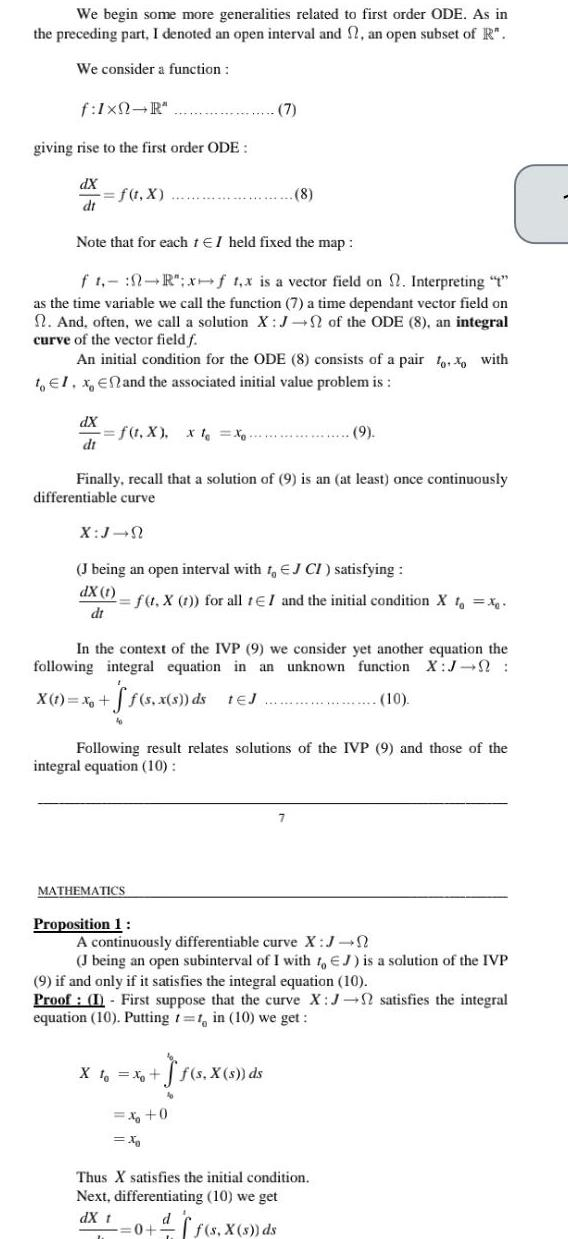Question:

# We begin some more generalities related to first order ODE

Last updated: 9/18/2023We begin some more generalities related to first order ODE As in the preceding part I denoted an open interval and 2 an open subset of R We consider a function f Ixn R giving rise to the first order ODE dX dt f t x dX dt Note that for each 1 EI held fixed the map ft R x f 1 x is a vector field on Interpreting as the time variable we call the function 7 a time dependant vector field on n And often we call a solution X J of the ODE 8 an integral curve of the vector field f An initial condition for the ODE 8 consists of a pair too with to El x En and the associated initial value problem is f t X x t Xo X J N Finally recall that a solution of 9 is an at least once continuously differentiable curve J being an open interval with 1 EJ CI satisfying dX 1 dt 7 f t X t for all tEI and the initial condition X to x MATHEMATICS 8 In the context of the IVP 9 we consider yet another equation the following integral equation in an unknown function X J X 1 Xo f s x s ds tEJ 10 Proposition 1 Following result relates solutions of the IVP 9 and those of the integral equation 10 J s X s ds 9 X to x A continuously differentiable curve X J J being an open subinterval of I with 1 EJ is a solution of the IVP 9 if and only if it satisfies the integral equation 10 Proof I First suppose that the curve X J satisfies the integral equation 10 Putting 1 1 in 10 we get 0 X f s X s ds Thus X satisfies the initial condition Next differentiating 10 we get dX t d 0 f s X